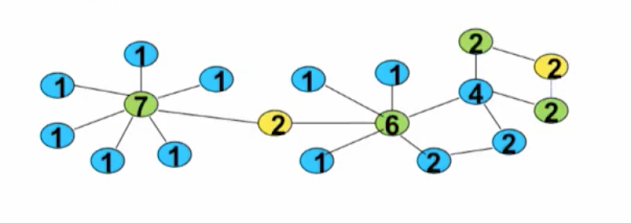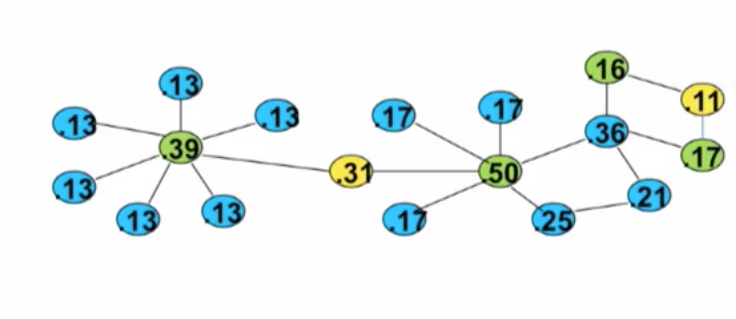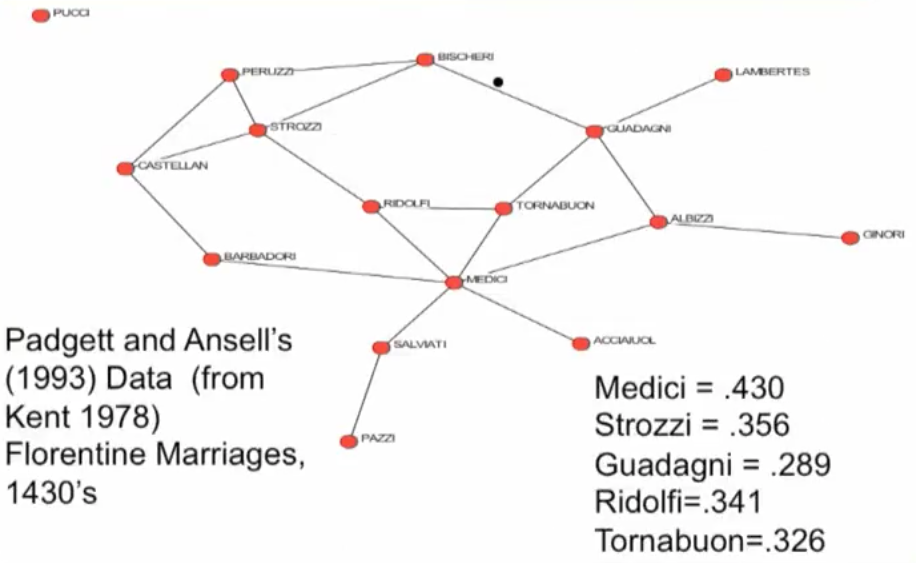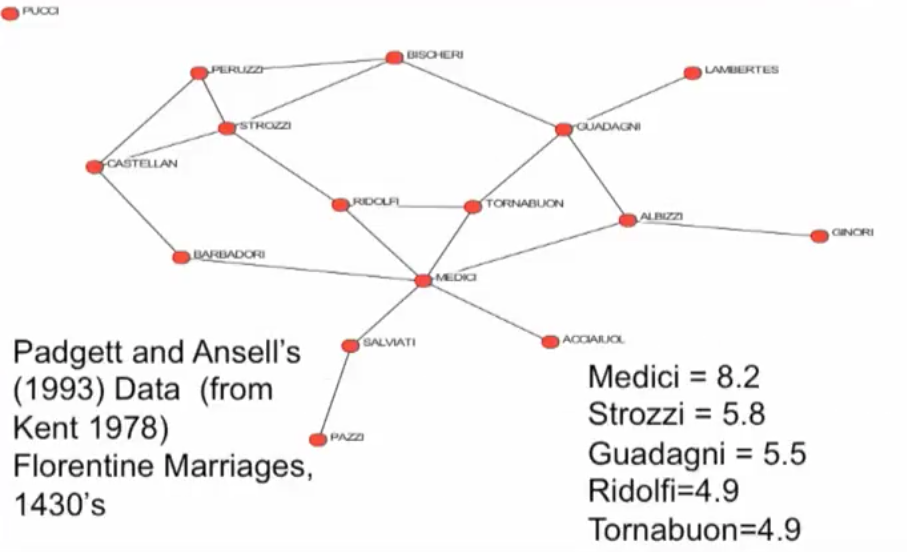# Position in Network¶

• How to describe individual characteristics?
• Degree
• Clustering
• Distance to other nodes
• Centrality, influence, power...???

# Degree Centrality?¶

• Failure of degree centrality to capture reach of a node:• More reach if connected to a 6 and 7 than a 2 and 2?we've looked at things like degree centrality it doesn't necessarily capture the importance of the node's friends, so you know, when we look at this picture, for instance... the, there, there's sort of two aspects to it. One is that we're, you know, this node might be much further, from a lot of nodes than this one. So, things like de, decay and closeness centrality can capture the fact that it's sort of out in the outskirts. But another thing is that when we look at the friends of this particular node. So if we look at the connections of this node they each have degree 2 themselves whereas this node's friends have degree 6 and 7. And so in some sense they are better connected than than this other node's friends. And so the idea of,of eigenvector centrality is that your importance comes from being connected to other important.

# Eigenvector Centrality¶

• If centrality is proportional to the sum of neighbors' centralities, then we're defining eigenvector centrality. So:

$C_i$ proportional to $\sum_{j:friend.of.i}C_j$

$C_i = a\sum_j g_{ij}C_j$

• $g_{ij} = 1$, then $c_j$ being counted
• $g_{ij} = 0$, then $c_j$ not counted
• we're just counting the centrality's of the friends you're connected to.

Basically what we have is that the vector C is equal to sum a times the matrix g times the vector C. And so this is what's known as an Eigenvector: $$C = agC$$

Now distinguishes more "influential" nodes# Prestige, Influence, Eigenvector - based Centrality¶

• Get value from connections to others, but proportional to their value

• Self-referential concept: $C_i^e(g) = a\sum_j g_{ij} C_j^e(g)$

• centrality is proportional to the summed centralities of neighbors
• $C_i^e(g) = a\sum_j g_{ij} C_j^e(g)$ $C^e(g) = agC^e(g)$

• $C^e(g)$ is an eigenvector - many possible solutions
• Look for one with laregest eigenvalue - will be nonnegative (Perron-Frobenius Theorem)
• normalize entries to sum to one

# Eigenvector Centrality¶# Centrality¶

• Concepts related to eigenvector centrality

• Google Page rank: score of a page is proportional to the sum of the scores of pages linked to it

• Random surfer model: start at some page on the web, randomly pick a link, follow it, repeat....# Bonacich Centrality¶

Builds on a measure by Katz

give each node a base value $ad_i(g)$ for some $a>0$, then add in all paths of length 1 from i to some j times b times j's base value, then add in all walks of length 2 from i to some j times $b^2$ j's base value...

$C^b(g) = ag1 + bgag1 + b^2g^2ag1...$

$= a(g1 + bg^21 + b^2g^31...)$

normalize $a$ to 1, need $small b$ to be finite

$C^b(g)= g1 + bg^21 + b^2g^31...$# Centrality, Four different things to measure¶

• Degree - connectedness
• Closeness, Decay - ease of reaching other nodes
• Betweenness - importance as an intermediary, connector
• Influence, Prestige, Eigenvectors - "not what you know, but who you know..."